Courses

# Alternating Current MCQ Level - 2

## 10 Questions MCQ Test Topic wise Tests for IIT JAM Physics | Alternating Current MCQ Level - 2

Description
This mock test of Alternating Current MCQ Level - 2 for Physics helps you for every Physics entrance exam. This contains 10 Multiple Choice Questions for Physics Alternating Current MCQ Level - 2 (mcq) to study with solutions a complete question bank. The solved questions answers in this Alternating Current MCQ Level - 2 quiz give you a good mix of easy questions and tough questions. Physics students definitely take this Alternating Current MCQ Level - 2 exercise for a better result in the exam. You can find other Alternating Current MCQ Level - 2 extra questions, long questions & short questions for Physics on EduRev as well by searching above.
QUESTION: 1

### An LCR series circuit with 100Ω resistance is connected to an AC source of 200V and angular frequency 300 radians per second. When only the capacitance is removed, the current lags the voltage by 60°. When only the inductance is removed, the current leads the voltage by 60°. Then the current and power dissipated in LCR circuit are respectively.

Solution:

When capacitance is removed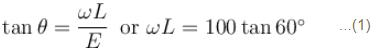when inductance is removed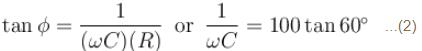From equation (1) and (2)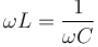So it is condition of resonance.
So  Z = R = 100Ω
I = V/R = 200/100= 2A
Power  P = I2R = 4 ×100 = 400 W
The correct answer is: 2A, 400Watt.

QUESTION: 2

### In the circuit diagram shown, XC = 100Ω, XL = 200Ω and  R =100Ω. The effective current through the source is :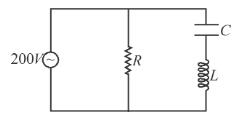Solution: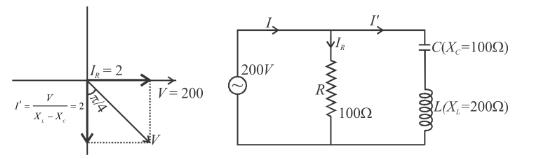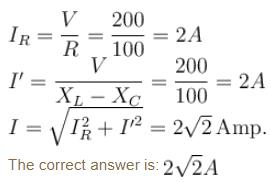QUESTION: 3

### An ac source of angular frequency ω is fed across a resistor R and a capacitor C in series. The current registered is I. If now the frequency of source is changed to ω/3 (but maintaining the same voltage), the current in the circuit is found to be halved. Then the ratio of reactance to resistance at the original frequency ω is

Solution:

According to given problem,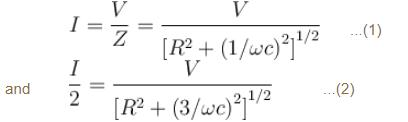Substituting the value of I from equation (1) in (2)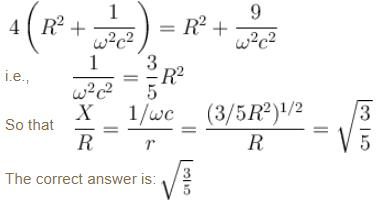QUESTION: 4

Current in an ac circuit is given by i = 3sin ωt + 4cos ωt, then :

Solution: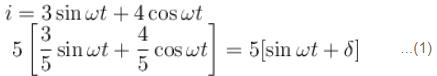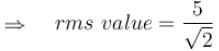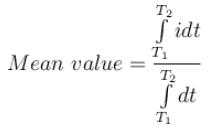∴   Initial value of time is not given hence the mean value will be different for various time intervals.
If voltage applied is  V = Vm sint ωt  then i given by equation (1) indicates that it is ahead by V by  δ   where  0 < δ < 90   which indicates that the circuit contains R and C.

The correct answer is: if voltage applied is V= Vmsin ωt,  then the circuit must be containing resistance and capacitance

QUESTION: 5

A current source sends a current i = i0 cos ωt. When connected across an unknown load gives a voltage output of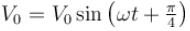across that load. Then voltage across the current source may be brought in phase with the current through it by: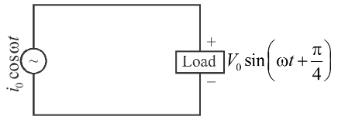Solution: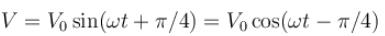Since V lags current, an inductor can bring it in phase with current.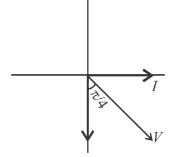The correct answer is: connecting an inductor in parallel with the load

QUESTION: 6

In series LCR circuit voltage drop across resistance is 8Volt, across inductor is 6Volt and across capacitor is 12Volt. Then:

Solution:

Since,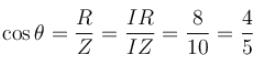(Also  cosθ  can never be greater than 1)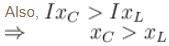In a LCR circuit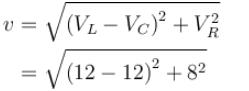V = 10; which is less than voltage drop across capacitor.
The correct answer is: none of these

QUESTION: 7

For a LCR series circuit with an AC source of angular frequency ω

Solution:

The circuit will have inductive nature if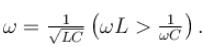Also if circuit has inductive nature will lag behind voltage.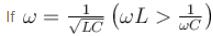the circuit will have resistance nature.
Power factor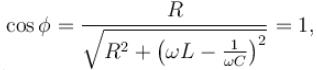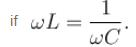Hence  true.

The correct answer is: power factor of circuit will be unity if capacitive reactance equals inductive reactance

QUESTION: 8

The secondary coil of an ideal step down transformer is delivering 500W power at 12.5A current. If the ratio of turns in the primary to the secondary is 5 : 1, then the current flowing in the primary coil will be :

Solution:

P = VI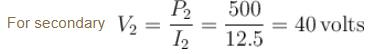For an ideal transformer (100% efficient)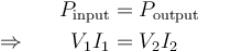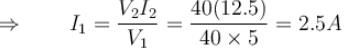QUESTION: 9

A bulb is rated at 100V, 100W, it can be treated as a resistor. Find out the inductance of an inductor (called choke coil) that should be connected in series with the bulb to operate the bulb at its rated power with the help of an ac source of 200V and 50Hz.

Solution:

From the rating of the bulb, the resistance of the bulb can be calculated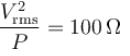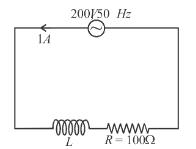For the bulb to be operated at its rated value the rms current through it should be 1A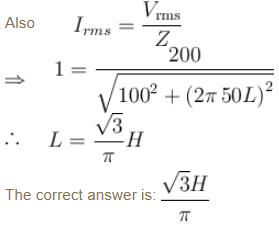QUESTION: 10

In a black box of unknown elements (L, C or R or any other combination) an AC voltage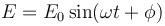is applied and current in the circuit was found to be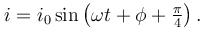Then the unknown elements in the box may be: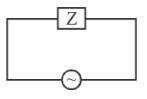Solution:

If we have all RL and C then I vs, E will be :
To obtain a leading phase difference of
if XL < XC and we use all RL and C in the circuit,
then the resultant graph will be :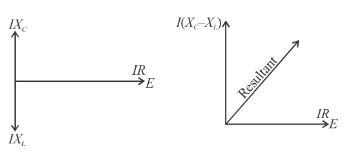which can give a leading phase difference of  π/4.
Similarly if we have only resistance and capacitor then we can obtain a phase difference of  π/4.
(leading) for suitable values of IXC and R. But we cannot obtain a leading phase difference of π/4. If we use only capacitor (phase difference of  π/2), or only (inductor and resistor) (phase difference of  π/2), or only resistor (phase difference of 0).

The correct answer is: either capacitor, resistor and inductor or only capacitor and resistor